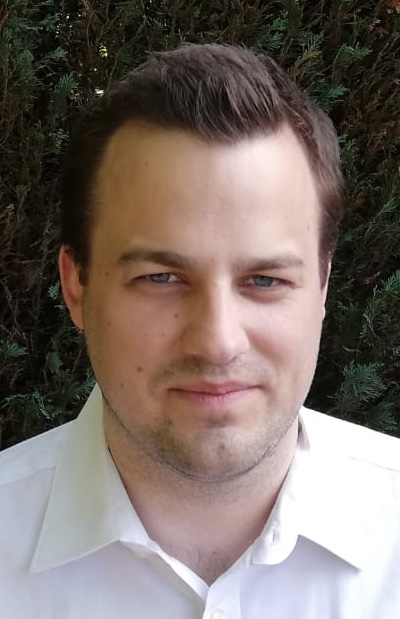# Julius Lohmann

Workgroup Prof. Dr. Benedikt Wirth
Institute for Computational and Applied Mathematics
University of Münster
Orléansring 10
D-48149 Münster

 Office: 120.026 Phone: +49 251 83-35133 Email: Please enable Javascript to see the email address## Research Interests

• Inverse Problems
• Optimal Transport
• Shape Optimization

## Ongoing Projects

I study a variant of the multimaterial transport problem, which was introduced by Andrea Marchese, Annalisa Massaccesi, and Riccardo Tione [2,3].

Optimization Problem (P): Let H:ℝm×n➝[0,∞) be a norm. Further, assume that μ=(μ1,...,μm) and ν=(ν1,...,νm) are the initial and final distributions (supported in some compact domain Ω in ℝn) of m different materials (with mass(μi)=mass(νi) for all i). Find an optimal multimaterial mass flux F between μ and ν with respect to H.

A multimaterial mass flux F between μ and ν can be modelled as a ℝm×n-valued Radon measure on Ω (with div(F)=μ-ν). The cost per distance to transport a material vector θ=(θ1,...,θm) in unit direction e is given by H(θ⊗e).

• The multimaterial transport problem in  (with cost per distance function ℤm➝[0,∞), which only allows for rectifiable F [Cor. on p. 265, 4]) was shown to be equivalent to a mass minimization problem on rectifiable 1-currents with coefficients in ℤm [Theorem 2.4, 3]. The authors of  introduced so-called calibrations as smooth ℝm-valued differential 1-forms that have to satisfy certain conditions related to the problem; these calibrations can be used to validate the optimality of candidate minimizers. Instead, we use a novel approach and introduce calibrations via primal-dual optimality conditions. More precisely, we study the case where H is induced (in a suitable sense) by a norm h:ℝm➝[0,∞) and consider the convex optimization problem (as in ) minF 𝕄h(F), where the minimization is over flat-1 chains F (with coefficients in ℝm) that satisfy ∂F=ν-μ. The functional 𝕄h denotes the h-mass. Using Fenchel-duality the optimality conditions of this problem and its dual yield our definition of calibrations. As a by-product we get exactly the true regularity of calibrations (neither too weak nor too strong). Moreover, the optimality conditions can be used to prove properties of optimal multimaterial mass fluxes F, and to provide an integral representation of 𝕄h(F) involving the cost function H (for rectifiable F this representation follows from [p. 268, 4]).

Joint work with: Bernhard Schmitzer and Benedikt Wirth.
• We provide a dynamic formulation of (P) (as time evolution of (multi-)mass and momentum) as well as some other properties of the induced distance dH. Moreover, we study a novel multiparticle tracking problem in which the trajectories of particles of m different types are to be determined. In particular, we aim to prove a sparsity property for the special case of Wasserstein-1 transport using the superposition principle in .

Joint work with: Marcello Carioni.

•  Ehsan Abedi, Zhenhao Li, and Timo Schultz. Absolutely continuous and BV-curves in 1-Wasserstein spaces. arXiv:2209.04268 [math.MG]. 2022.
 Andrea Marchese, Annalisa Massaccesi, Salvatore Stuvard, and Riccardo Tione. A multi-material transport problem with arbitrary marginals. Calculus of Variations and Partial Differential Equations 60 (2021) Article 88.
 Andrea Marchese, Annalisa Massaccesi, and Riccardo Tione. A Multimaterial Transport Problem and its Convex Relaxation via Rectifiable G-currents. SIAM Journal on Mathematical Analysis 51:3 (2019) 1965-1998.
 Brian White. The deformation theorem for flat chains. Acta Mathematica 183:2 (1999) 255-271.

## Publications

• Julius Lohmann, Bernhard Schmitzer, and Benedikt Wirth. Duality in branched transport and urban planning. Applied Mathematics and Optimization 86:3 (2022) Article 45. [Preprint] [Version of Record]
• Julius Lohmann, Bernhard Schmitzer, and Benedikt Wirth. Formulation of branched transport as geometry optimization. Journal de Mathématiques Pures et Appliquées 163 (2022) 739-779. [Preprint] [Version of Record]

## Education

• 2019-2023 PhD student at University of Münster
• 2018-2019 Research assistant at FAU Erlangen-Nürnberg
• 2015-2016 Semester abroad at Stockholm University
• 2014-2017 MSc Mathematics (minor Computer Science) at FAU Erlangen-Nürnberg
• 2011-2014 BSc Mathematics (minor Computer Science) at FAU Erlangen-Nürnberg